# Analysis of the 2016 US Presidential Election

### Chapter 5.5: Model selection criteria

The data for this analysis come from Tony McGovern. The response variable, $$Y_i$$, is the percentage change in Republican (GOP) support from 2012 to 2016, i.e., $100\left(\frac{\mbox{% in 2016}}{\mbox{% in 2012}}-1\right),$ in county $$i=1,…,n$$.

The $$p=10$$ covariates $$X_{ij}$$ are county-level census variables obtained from Kaggle are:

• Population, percent change - April 1, 2010 to July 1, 2014
• Persons 65 years and over, percent, 2014
• Black or African American alone, percent, 2014
• Hispanic or Latino, percent, 2014
• High school graduate or higher, percent of persons age 25+, 2009-2013
• Bachelor's degree or higher, percent of persons age 25+, 2009-2013
• Homeownership rate, 2009-2013
• Median value of owner-occupied housing units, 2009-2013
• Median household income, 2009-2013
• Persons below poverty level, percent, 2009-2013

For a county in state $$s$$, we assume the linear model $Y_i=\beta_{0s}+\sum_{j=1}^pX_i\beta_{sj} + \varepsilon_i,$ where $$\beta_{js}$$ is the effect of covariate $$j$$ in state $$s$$. We compare three models for the $$\beta_{js}$$

1. Constant slopes: $$\beta_{js} \equiv \beta_j$$ for all counties
2. Varying slopes with uninformative priors: $$\beta_{js} \sim\mbox{Normal}(0,100)$$
3. Varying slopes with informative priors: $$\beta_{js} \sim\mbox{Normal}(\mu_j,\sigma_j^2)$$

In the third model, the means $$\mu_j$$ and variances $$\sigma_j^2$$ are given prior and estimated from the data, therefore information is pooled across states via the prior. The three methods are compared using DIC, and final results are compared across models.

## Load the data

 load("election_2008_2016.RData")
X     <- scale(X)    # Standardize the covariates
X     <- cbind(1,X)
short <- c("Intercept", "Pop change", "65+","African American",
"Hispanic","HS grad","Bachelor's",
"Homeownership rate","Home value",
"Median income", "Poverty")
names <- c("Intercept", as.character(names[1:11,2]))
colnames(X) <- short

# Define a function to make county maps
county_plot <- function(fips,Y,main="",units=""){
library(choroplethr)
temp  <- as.data.frame(list(region=fips,value=Y))
suppressWarnings(county_choropleth(temp,title=main,legend=units))
}
county_plot(fips,Y,main="Percent increase in GOP support",units="")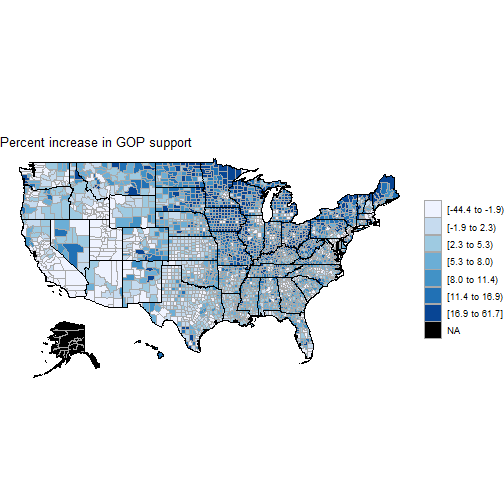county_plot(fips,X[,7],main=names,units="")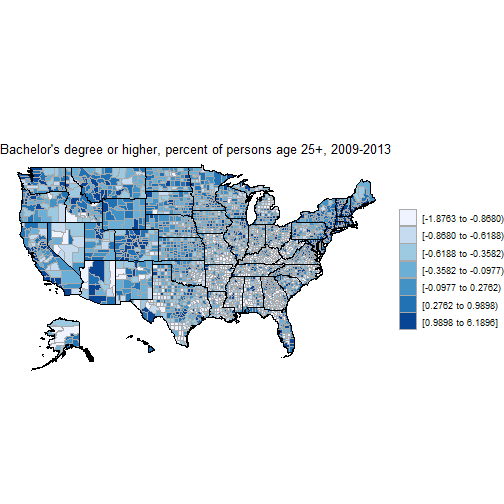# Remove AK, HI and DC due to missing data
state <- as.character(all_dat[,3])
AKHI  <- state=="AK" | state=="HI" | state=="DC"
fips  <- fips[!AKHI]
Y     <- Y[!AKHI]
X     <- X[!AKHI,]
state <- state[!AKHI]

# Assign a numeric id to the counties in each state
st    <- unique(state)
id    <- rep(NA,length(Y))
for(j in 1:48){
id[state==st[j]]<-j
}

n <- length(Y) # number of counties
N <- 48        # number of states
p <- ncol(X)   # number of covariates

set.seed(0820)
iters <- 50000
burn  <- 10000


## (1) Constant slopes

 model1_string <- "model{

# Likelihood
for(i in 1:n){
Y[i]   ~ dnorm(mu[i],taue)
mu[i] <- inprod(X[i,],beta[])
}
# Priors
for(j in 1:p){beta[j] ~ dnorm(0,0.01)}
taue ~ dgamma(0.1,0.1)
sig <- 1/sqrt(taue)

# WAIC calculations
for(i in 1:n){
like[i]    <- dnorm(Y[i],mu[i],taue)
}
}"

library(rjags)

# Load the model
dat    <- list(Y=Y,n=n,X=X,p=p)
init   <- list(beta=rep(0,p))
model1 <- jags.model(textConnection(model1_string),n.chains=2,
inits=init,data = dat,quiet=TRUE)
# Generate samples
update(model1, burn, progress.bar="none")
samp1   <- coda.samples(model1,
variable.names=c("beta"),
n.iter=iters, progress.bar="none")

# Compile results
ESS1    <- effectiveSize(samp1)
out1    <- summary(samp1)$quantiles rownames(out1)<-short # Compute DIC dic1 <- dic.samples(model1,n.iter=iters,progress.bar="none") # Compute WAIC waic1 <- coda.samples(model1, variable.names=c("like"), n.iter=iters, progress.bar="none") like1 <- waic1[] fbar1 <- colMeans(like1) P1 <- sum(apply(log(like1),2,var)) WAIC1 <- -2*sum(log(fbar1))+2*P1  ## (2) Slopes as fixed effects  model2_string <- "model{ # Likelihood for(i in 1:n){ Y[i] ~ dnorm(mnY[i],taue) mnY[i] <- inprod(X[i,],beta[id[i],]) } # Slopes for(j in 1:p){for(i in 1:N){ beta[i,j] ~ dnorm(0,0.01) }} # Priors taue ~ dgamma(0.1,0.1) # WAIC calculations for(i in 1:n){ like[i] <- dnorm(Y[i],mnY[i],taue) } }" library(rjags) # Load the model dat <- list(Y=Y,n=n,N=N,X=X,p=p,id=id) init <- list(beta=matrix(0,N,p)) model2 <- jags.model(textConnection(model2_string),n.chains=2, inits=init,data = dat,quiet=TRUE) # Generate samples update(model2, burn, progress.bar="none") samp2 <- coda.samples(model2, variable.names=c("beta"), n.iter=iters, progress.bar="none") # Compile results ESS2 <- effectiveSize(samp2) sum <- summary(samp2)$stat
post_mn2 <- matrix(sum[,1],N,p)
post_sd2 <- matrix(sum[,2],N,p)

# Compute DIC
dic2   <- dic.samples(model2,n.iter=iters,progress.bar="none")

# Compute WAIC
waic2   <- coda.samples(model2,
variable.names=c("like"),
n.iter=iters, progress.bar="none")
like2   <- waic2[]
fbar2   <- colMeans(like2)
P2      <- sum(apply(log(like2),2,var))
WAIC2   <- -2*sum(log(fbar2))+2*P2


## (3) Slopes as random effects

 model3_string <- "model{

# Likelihood
for(i in 1:n){
Y[i]    ~ dnorm(mnY[i],taue)
mnY[i] <- inprod(X[i,],beta[id[i],])
}

# Random slopes
for(j in 1:p){
for(i in 1:N){
beta[i,j] ~ dnorm(mu[j],taub[j])
}
mu[j]    ~ dnorm(0,0.01)
taub[j]  ~ dgamma(0.1,0.1)
}

# Priors
taue ~ dgamma(0.1,0.1)

# WAIC calculations
for(i in 1:n){
like[i]    <- dnorm(Y[i],mnY[i],taue)
}
}"

library(rjags)

# Load the model
dat    <- list(Y=Y,n=n,N=N,X=X,p=p,id=id)
init   <- list(beta=matrix(0,N,p))
model3 <- jags.model(textConnection(model3_string),n.chains=2,
inits=init,data = dat,quiet=TRUE)

# Generate samples
update(model3, burn, progress.bar="none")
samp3  <- coda.samples(model3,
variable.names=c("beta"),
n.iter=iters, progress.bar="none")

# Compile results
ESS3     <- effectiveSize(samp3)
sum      <- summary(samp3)\$stat
post_mn3 <- matrix(sum[,1],N,p)
post_sd3 <- matrix(sum[,2],N,p)

# Compute DIC
dic3    <- dic.samples(model3,n.iter=iters,progress.bar="none")

# Compute WAIC
waic3   <- coda.samples(model3,
variable.names=c("like"),
n.iter=iters, progress.bar="none")
like3   <- waic3[]
fbar3   <- colMeans(like3)
P3      <- sum(apply(log(like3),2,var))
WAIC3   <- -2*sum(log(fbar3))+2*P3


## Check convergence

 ESS1

##   beta   beta   beta   beta   beta   beta   beta
## 99338.223 53652.963 18626.686 41660.287 30666.972 16547.198 15945.338
##   beta   beta  beta  beta
## 24268.764 17264.099  8718.938 12147.265

 hist(ESS2)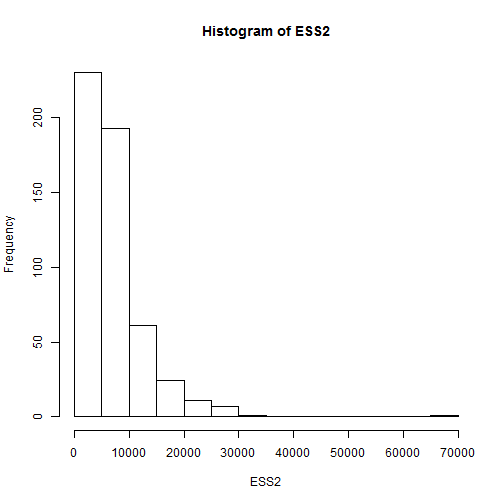hist(ESS3)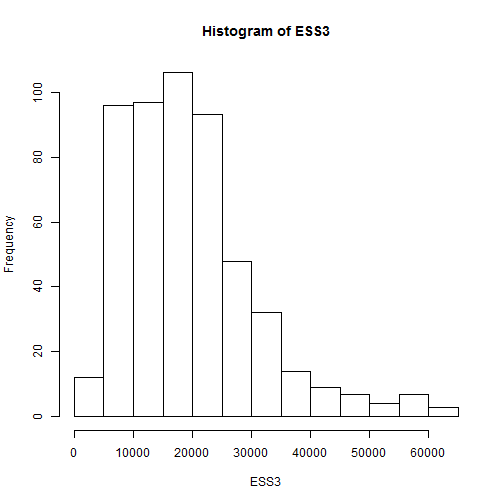Summary: The effective sample size is large for all parameters and all models, therefore it seems the MCMC algorithm has converged.

## Summarize the non-spatial model

 kable(round(out1,2))

2.5% 25% 50% 75% 97.5%
Intercept 6.41 6.58 6.67 6.76 6.93
Pop change -1.46 -1.25 -1.14 -1.03 -0.82
65+ 0.53 0.79 0.93 1.06 1.32
African American -1.89 -1.67 -1.56 -1.45 -1.23
Hispanic -2.40 -2.18 -2.06 -1.95 -1.72
HS grad 1.24 1.57 1.75 1.92 2.25
Bachelor's -6.71 -6.37 -6.19 -6.01 -5.66
Homeownership rate -0.38 -0.13 0.01 0.15 0.41
Home value -1.98 -1.68 -1.52 -1.36 -1.05
Median income 1.14 1.62 1.87 2.12 2.60
Poverty 0.91 1.28 1.47 1.66 2.03

Summary: All but one (home ownership rate) of the covariates have 95% interval that excludes zero. GOP support tended to increase in counties with

• decreasing population
• high proportion of seniors and high school graduates
• low proportions of African Americans and Hispanics
• High income but low home value
• High poverty rate

## Compare models with DIC

 dic1

## Mean deviance:  21300
## penalty 11.98
## Penalized deviance: 21312

 dic2

## Mean deviance:  18484
## penalty 454.9
## Penalized deviance: 18938

 dic3

## Mean deviance:  18604
## penalty 237.5
## Penalized deviance: 18842


Summary: The first model with constant slopes is the simplest but fits the data poorly and thus has highest DIC. The second model with different slopes in each state has the best fit (smallest mean deviance), but is too complicated and has large $$p_D$$. The final model has fairly small mean deviance and $$p_D$$, and thus balances fit and complexity to give the smallest DIC.

## Compare models with WAIC

 WAIC1; P1

##  21334.86

##  20.02579

 WAIC2; P2

##  18970.65

##  405.3173

 WAIC3; P3

##  18910.91

##  259.5478


Summary: WAIC agrees with DIC. Both prefer Model 3 with the regression coefficients treated as random effects.

## Explore the results of the final model

 boxplot(post_mn3)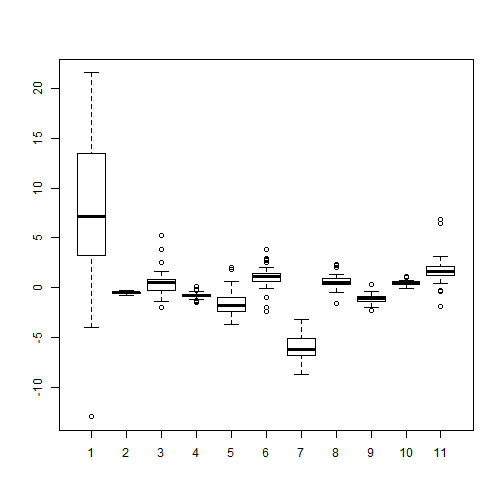Summary: The effect of the proportion of college graduates varies the most across states

## Explore the three estimate of the effects of college graduates

 # Posterior mean
county_plot(fips,post_mn3[id,7],
main="Proportion of college graduates - posterior mean")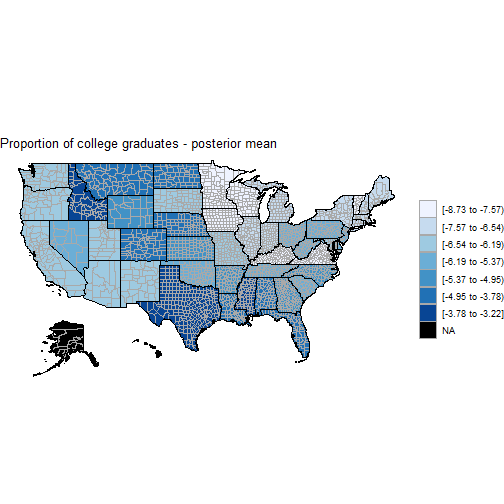# Posterior sd
county_plot(fips,post_sd3[id,7],
main="Proportion of college graduates - posterior SD")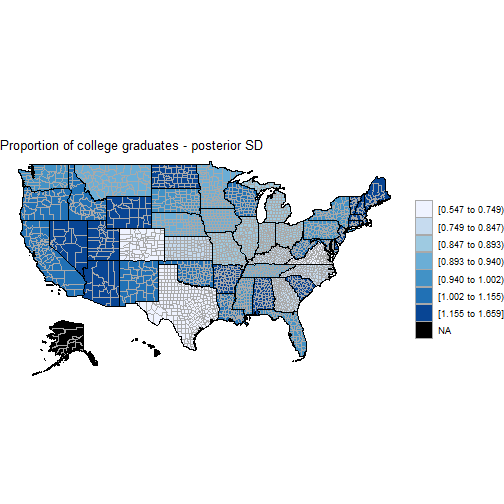Summary: The effect of the proportion of college graduates is the strongest (most negative) in the midwest.